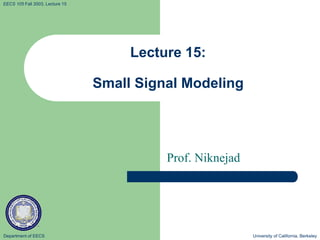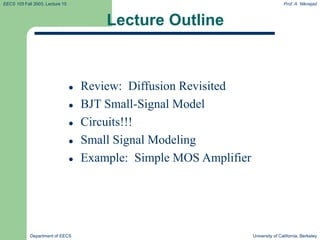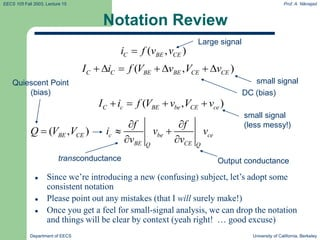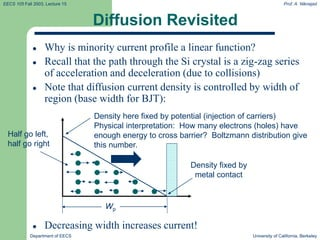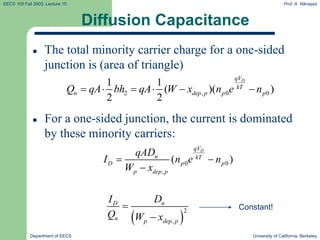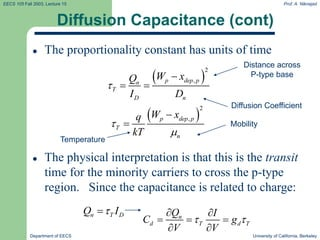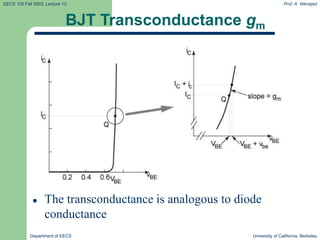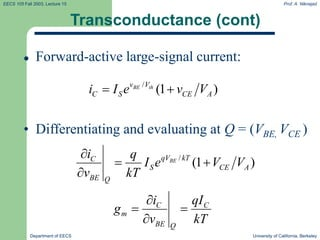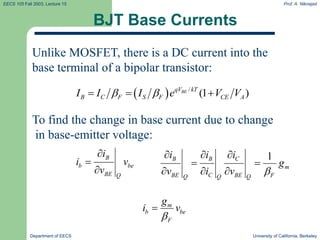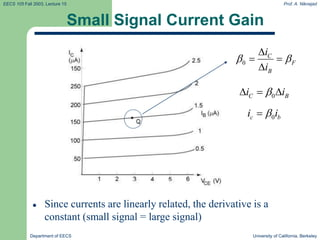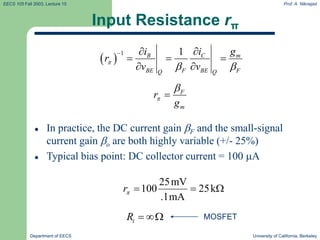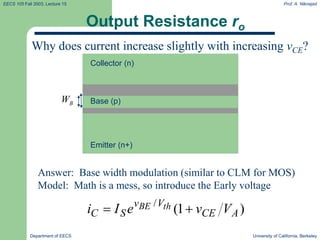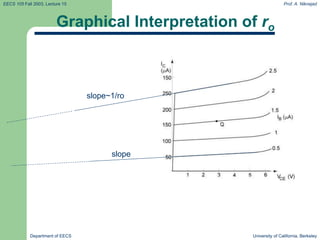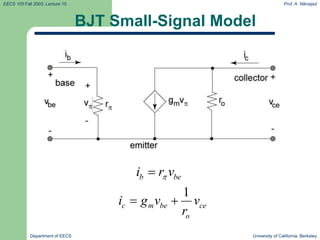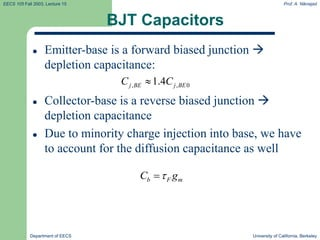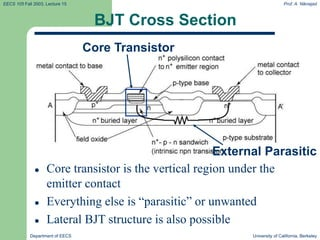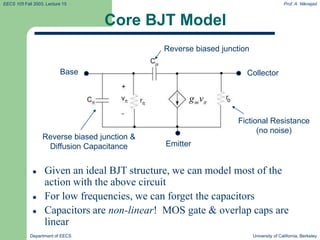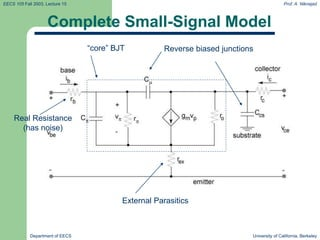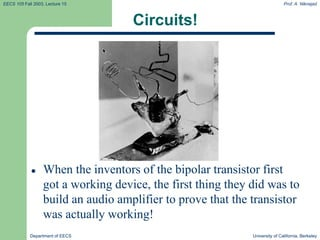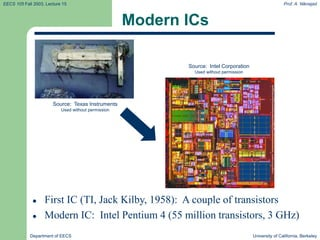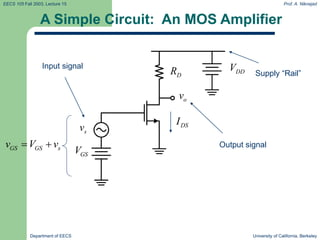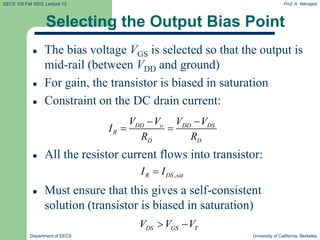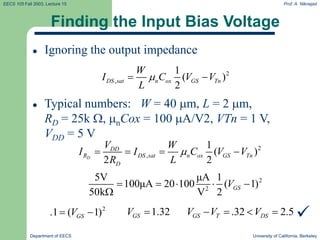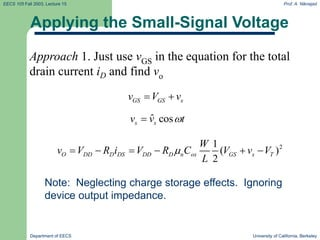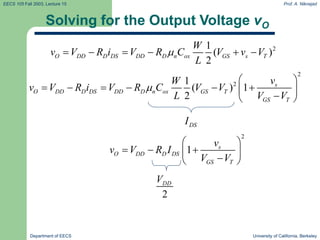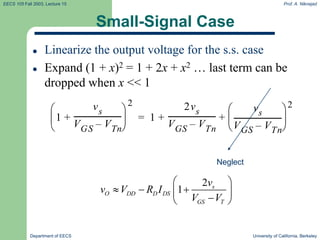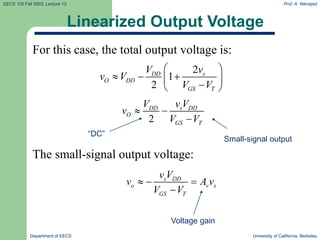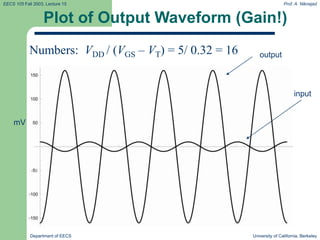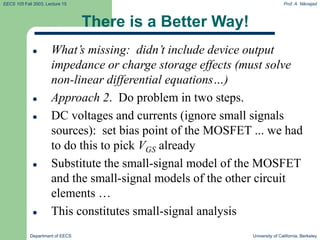1 of 29

### Lecture15.ppt

1. Department of EECS University of California, Berkeley EECS 105 Fall 2003, Lecture 15 Lecture 15: Small Signal Modeling Prof. Niknejad
2. EECS 105 Fall 2003, Lecture 15 Prof. A. Niknejad Department of EECS University of California, Berkeley Lecture Outline  Review: Diffusion Revisited  BJT Small-Signal Model  Circuits!!!  Small Signal Modeling  Example: Simple MOS Amplifier
3. EECS 105 Fall 2003, Lecture 15 Prof. A. Niknejad Department of EECS University of California, Berkeley Notation Review  Since we’re introducing a new (confusing) subject, let’s adopt some consistent notation  Please point out any mistakes (that I will surely make!)  Once you get a feel for small-signal analysis, we can drop the notation and things will be clear by context (yeah right! … good excuse) ( , ) C BE CE i f v v  Large signal ( , ) C C BE BE CE CE I i f V v V v        small signal DC (bias) ( , ) C c BE be CE ce I i f V v V v     small signal (less messy!) c be ce BE CE Q Q f f i v v v v       transconductance Output conductance ( , ) BE CE Q V V  Quiescent Point (bias)
4. EECS 105 Fall 2003, Lecture 15 Prof. A. Niknejad Department of EECS University of California, Berkeley Diffusion Revisited  Why is minority current profile a linear function?  Recall that the path through the Si crystal is a zig-zag series of acceleration and deceleration (due to collisions)  Note that diffusion current density is controlled by width of region (base width for BJT):  Decreasing width increases current! Density here fixed by potential (injection of carriers) Physical interpretation: How many electrons (holes) have enough energy to cross barrier? Boltzmann distribution give this number. Wp Density fixed by metal contact Half go left, half go right
5. EECS 105 Fall 2003, Lecture 15 Prof. A. Niknejad Department of EECS University of California, Berkeley Diffusion Capacitance  The total minority carrier charge for a one-sided junction is (area of triangle)  For a one-sided junction, the current is dominated by these minority carriers: 2 , 0 0 1 1 ( )( ) 2 2 D qV kT n dep p p p Q qA bh qA W x n e n       0 0 , ( ) D qV n kT D p p p dep p qAD I n e n W x      2 , n D n p dep p D I Q W x   Constant!
6. EECS 105 Fall 2003, Lecture 15 Prof. A. Niknejad Department of EECS University of California, Berkeley Diffusion Capacitance (cont)  The proportionality constant has units of time  The physical interpretation is that this is the transit time for the minority carriers to cross the p-type region. Since the capacitance is related to charge:   2 , p dep p n T D n W x Q I D     n T D Q I   n d T d T Q I C g V V          Diffusion Coefficient Distance across P-type base   2 , p dep p T n W x q kT     Mobility Temperature
7. EECS 105 Fall 2003, Lecture 15 Prof. A. Niknejad Department of EECS University of California, Berkeley BJT Transconductance gm  The transconductance is analogous to diode conductance
8. EECS 105 Fall 2003, Lecture 15 Prof. A. Niknejad Department of EECS University of California, Berkeley Transconductance (cont)  Forward-active large-signal current: / (1 ) BE th v V C S CE A i I e v V   • Differentiating and evaluating at Q = (VBE, VCE ) / (1 ) BE qV kT C S CE A BE Q i q I e V V v kT     C C m BE Q i qI g v kT    
9. EECS 105 Fall 2003, Lecture 15 Prof. A. Niknejad Department of EECS University of California, Berkeley BJT Base Currents Unlike MOSFET, there is a DC current into the base terminal of a bipolar transistor:   / (1 ) BE qV kT B C F S F CE A I I I e V V      To find the change in base current due to change in base-emitter voltage: 1 B B C m BE C BE F Q Q Q i i i g v i v          B b be BE Q i i v v    m b be F g i v  
10. EECS 105 Fall 2003, Lecture 15 Prof. A. Niknejad Department of EECS University of California, Berkeley Small Signal Current Gain 0 C F B i i        Since currents are linearly related, the derivative is a constant (small signal = large signal) 0 C B i i     0 c b i i  
11. EECS 105 Fall 2003, Lecture 15 Prof. A. Niknejad Department of EECS University of California, Berkeley Input Resistance rπ   1 1 C m B BE F BE F Q Q i g i r v v             In practice, the DC current gain F and the small-signal current gain o are both highly variable (+/- 25%)  Typical bias point: DC collector current = 100 A F m r g    25mV 100 25k .1mA r    i R   MOSFET
12. EECS 105 Fall 2003, Lecture 15 Prof. A. Niknejad Department of EECS University of California, Berkeley Output Resistance ro Why does current increase slightly with increasing vCE? Answer: Base width modulation (similar to CLM for MOS) Model: Math is a mess, so introduce the Early voltage ) 1 ( / A CE V v S C V v e I i th BE   Base (p) Emitter (n+) Collector (n) B W
13. EECS 105 Fall 2003, Lecture 15 Prof. A. Niknejad Department of EECS University of California, Berkeley Graphical Interpretation of ro slope~1/ro slope
14. EECS 105 Fall 2003, Lecture 15 Prof. A. Niknejad Department of EECS University of California, Berkeley BJT Small-Signal Model b be i r v   1 c m be ce o i g v v r  
15. EECS 105 Fall 2003, Lecture 15 Prof. A. Niknejad Department of EECS University of California, Berkeley BJT Capacitors  Emitter-base is a forward biased junction  depletion capacitance:  Collector-base is a reverse biased junction  depletion capacitance  Due to minority charge injection into base, we have to account for the diffusion capacitance as well , , 0 1.4 j BE j BE C C  b F m C g  
16. EECS 105 Fall 2003, Lecture 15 Prof. A. Niknejad Department of EECS University of California, Berkeley BJT Cross Section  Core transistor is the vertical region under the emitter contact  Everything else is “parasitic” or unwanted  Lateral BJT structure is also possible Core Transistor External Parasitic
17. EECS 105 Fall 2003, Lecture 15 Prof. A. Niknejad Department of EECS University of California, Berkeley Core BJT Model  Given an ideal BJT structure, we can model most of the action with the above circuit  For low frequencies, we can forget the capacitors  Capacitors are non-linear! MOS gate & overlap caps are linear m g v Base Collector Emitter Reverse biased junction Reverse biased junction & Diffusion Capacitance Fictional Resistance (no noise)
18. EECS 105 Fall 2003, Lecture 15 Prof. A. Niknejad Department of EECS University of California, Berkeley Complete Small-Signal Model Reverse biased junctions “core” BJT External Parasitics Real Resistance (has noise)
19. EECS 105 Fall 2003, Lecture 15 Prof. A. Niknejad Department of EECS University of California, Berkeley Circuits!  When the inventors of the bipolar transistor first got a working device, the first thing they did was to build an audio amplifier to prove that the transistor was actually working!
20. EECS 105 Fall 2003, Lecture 15 Prof. A. Niknejad Department of EECS University of California, Berkeley Modern ICs  First IC (TI, Jack Kilby, 1958): A couple of transistors  Modern IC: Intel Pentium 4 (55 million transistors, 3 GHz) Source: Texas Instruments Used without permission Source: Intel Corporation Used without permission
21. EECS 105 Fall 2003, Lecture 15 Prof. A. Niknejad Department of EECS University of California, Berkeley A Simple Circuit: An MOS Amplifier DS I GS V s v D R DD V GS GS s v V v   o v Input signal Output signal Supply “Rail”
22. EECS 105 Fall 2003, Lecture 15 Prof. A. Niknejad Department of EECS University of California, Berkeley Selecting the Output Bias Point  The bias voltage VGS is selected so that the output is mid-rail (between VDD and ground)  For gain, the transistor is biased in saturation  Constraint on the DC drain current:  All the resistor current flows into transistor:  Must ensure that this gives a self-consistent solution (transistor is biased in saturation) DD o DD DS R D D V V V V I R R     , R DS sat I I  DS GS T V V V  
23. EECS 105 Fall 2003, Lecture 15 Prof. A. Niknejad Department of EECS University of California, Berkeley Finding the Input Bias Voltage  Ignoring the output impedance  Typical numbers: W = 40 m, L = 2 m, RD = 25k, nCox = 100 A/V2, VTn = 1 V, VDD = 5 V 2 , 1 ( ) 2 DS sat n ox GS Tn W I C V V L    2 , 1 ( ) 2 2 D DD R DS sat n ox GS Tn D V W I I C V V R L      2 2 5V μA 1 100μA 20 100 ( 1) 50k V 2 GS V       2 .1 ( 1) GS V   1.32 GS V  .32 2.5 GS T DS V V V     
24. EECS 105 Fall 2003, Lecture 15 Prof. A. Niknejad Department of EECS University of California, Berkeley Applying the Small-Signal Voltage Approach 1. Just use vGS in the equation for the total drain current iD and find vo GS GS s v V v   ˆ cos s s v v t   2 1 ( ) 2 O DD D DS DD D n ox GS s T W v V R i V R C V v V L        Note: Neglecting charge storage effects. Ignoring device output impedance.
25. EECS 105 Fall 2003, Lecture 15 Prof. A. Niknejad Department of EECS University of California, Berkeley Solving for the Output Voltage vO 2 1 ( ) 2 O DD D DS DD D n ox GS s T W v V R i V R C V v V L        2 2 1 ( ) 1 2 s O DD D DS DD D n ox GS T GS T v W v V R i V R C V V L V V               DS I 2 1 s O DD D DS GS T v v V R I V V           2 DD V
26. EECS 105 Fall 2003, Lecture 15 Prof. A. Niknejad Department of EECS University of California, Berkeley Small-Signal Case  Linearize the output voltage for the s.s. case  Expand (1 + x)2 = 1 + 2x + x2 … last term can be dropped when x << 1 1 vs VGS VTn – ------------------------- - +       2 1 2vs VGS VTn – ------------------------- - vs VGS VTn – ------------------------- -      2 + + = Neglect 2 1 s O DD D DS GS T v v V R I V V          
27. EECS 105 Fall 2003, Lecture 15 Prof. A. Niknejad Department of EECS University of California, Berkeley Linearized Output Voltage For this case, the total output voltage is: The small-signal output voltage: 2 1 2 s DD O DD GS T v V v V V V           2 s DD DD O GS T v V V v V V    “DC” Small-signal output s DD o v s GS T v V v A v V V     Voltage gain
28. EECS 105 Fall 2003, Lecture 15 Prof. A. Niknejad Department of EECS University of California, Berkeley Plot of Output Waveform (Gain!) Numbers: VDD / (VGS – VT) = 5/ 0.32 = 16 output input mV
29. EECS 105 Fall 2003, Lecture 15 Prof. A. Niknejad Department of EECS University of California, Berkeley There is a Better Way!  What’s missing: didn’t include device output impedance or charge storage effects (must solve non-linear differential equations…)  Approach 2. Do problem in two steps.  DC voltages and currents (ignore small signals sources): set bias point of the MOSFET ... we had to do this to pick VGS already  Substitute the small-signal model of the MOSFET and the small-signal models of the other circuit elements …  This constitutes small-signal analysis